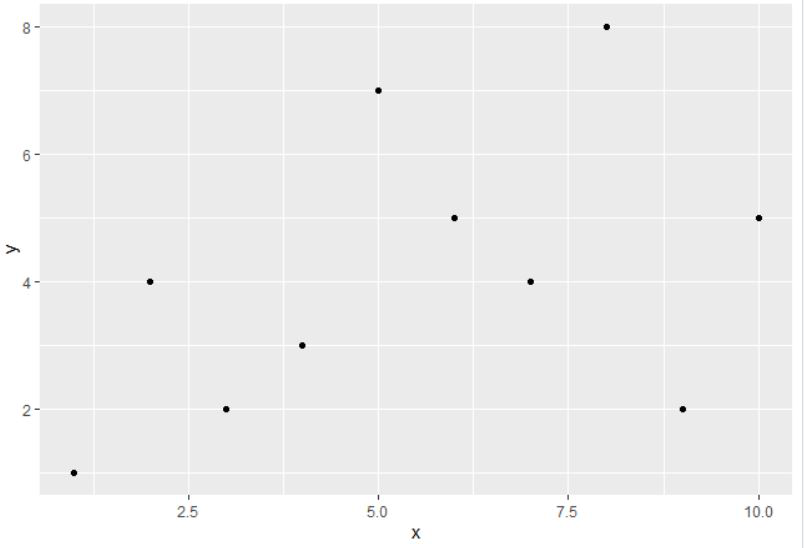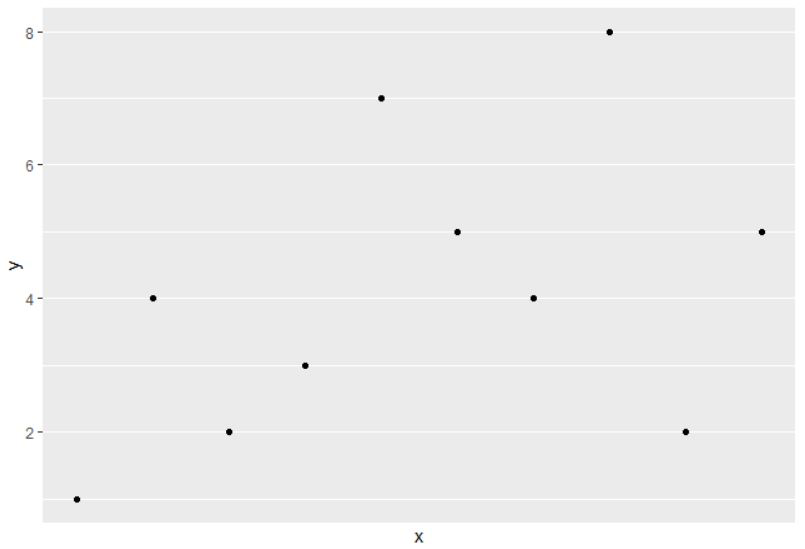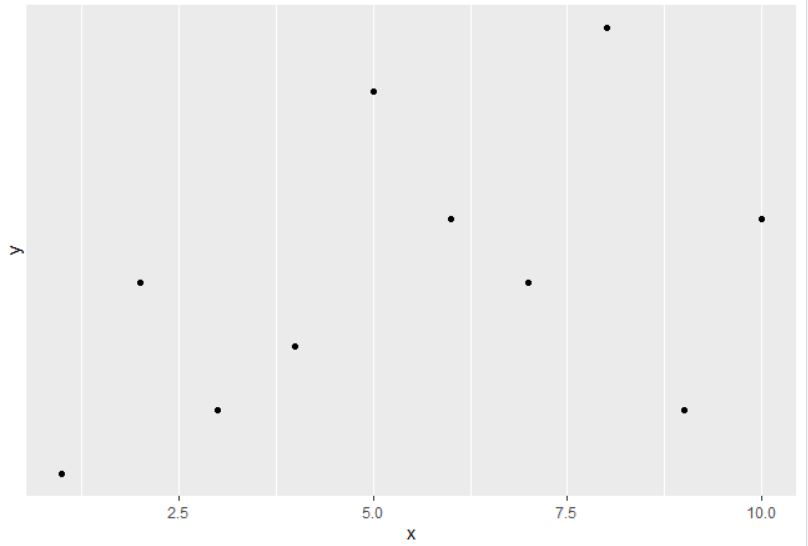Open in App
Not now

# Remove Vertical or Horizontal Gridlines in ggplot2 Plot in R

• Difficulty Level : Easy
• Last Updated : 14 Sep, 2021

In this article, we are going to learn how to remove Vertical or Horizontal Gridlines in the background of a ggplot2 Plot in R programming language.

To understand how to remove Vertical or Horizontal Gridlines, first import all the required libraries to the working space and create a dataframe. Let us first create a regular plot so that the difference is apparent.

Example: default plot

## R

 `library``(``"ggplot2"``) `` ` `# Store 10 entries of data in data frame``A <- ``data.frame``(x = 1:10, y = ``c``(1,4,2,3,7,5,4,8,2,5))`` ` `# A ggplot2 plot with gridlines``p <- ``ggplot``(A, ``aes``(x, y)) + ``geom_point``()`` ` `# Display the plot``p`

Output:Now for removing gridlines, separate functions are added while creating a plot. In ggplot2, scales control how the data is mapped into aesthetics. When we provide some data, it takes the data and converts it into something visual for us like position, size, color, or shape.

Scales toolbox can have different attributes like the following two main types:

• Position scales and axes
• Colour scales and legend

In order to remove gridlines, we are going to focus on position scales. There are two position scales in a plot corresponding to x and y aesthetics. Two most common types of continuous position scales are the default scale_x_continuous() and scale_y_continuous() functions. We are going to use these functions to remove the gridlines.

To remove vertical grid lines scale_x_continuous() function is passed with the breaks parameter as NULL.

Syntax:

scale_x_continuous(breaks =NULL )

Example: Removing vertical gridlines

## R

 `library``(``"ggplot2"``) `` ` `# Store 10 entries of data in data frame``A <- ``data.frame``(x = 1:10, y = ``c``(1,4,2,3,7,5,4,8,2,5))`` ` `# A ggplot2 plot with gridlines``p <- ``ggplot``(A, ``aes``(x, y)) + ``geom_point``()`` ` `# Remove Vertical Gridlines ``p + ``scale_x_continuous``(breaks = ``NULL``)`

Output:Similarly for removing horizontal gridlines break parameter of scale_y_continuous() is set to NULL.

Example: Removing horizontal lines

## R

 `library``(``"ggplot2"``) `` ` `# Store 10 entries of data in data frame``A <- ``data.frame``(x = 1:10, y = ``c``(1,4,2,3,7,5,4,8,2,5))`` ` `# A ggplot2 plot with gridlines``p <- ``ggplot``(A, ``aes``(x, y)) + ``geom_point``()`` ` `# Remove Horizontal Gridlines``p + ``scale_y_continuous``(breaks = ``NULL``)`

Output:My Personal Notes arrow_drop_up